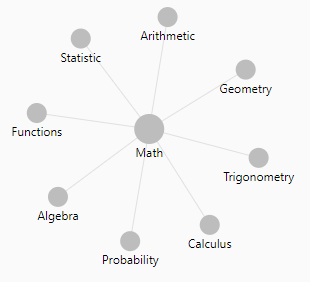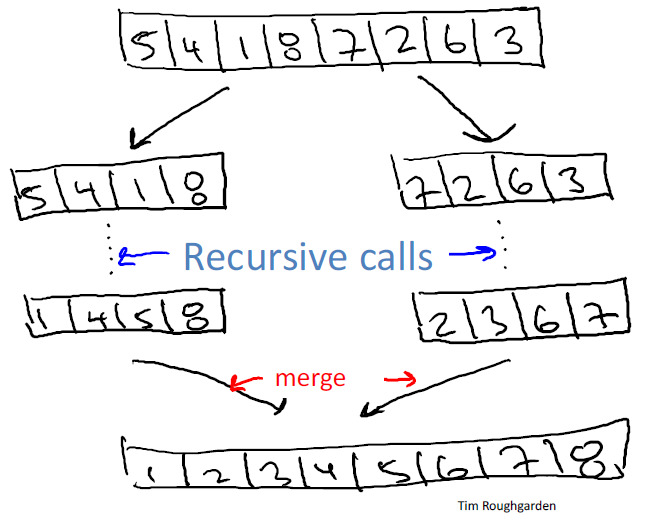# Mathematics - Logarithm Function (log)

$log_b(n)$ is the number (#) of times you divide n by b until you get down to 1.

If you see log, you must think trees

## Example

With the base 2 where $log_2 n$ is the # of times you divide n by 2 until you get down to 1.

You keep repeating dividing by two and you count how many times you divide by two until you get a number that drops below one

$$\begin{array}{rrl} log_2 (42) = 5.39 &&&\\ 1- & 42/2 & = & 21 \\ 2- & 21/2 & = & 10.5 \\ 3- & 10.5 / 2 & = & 5.25 \\ 4- & 5.25/2 & = & 2.625 \\ 5- & 2.625/2 & = & 1.3125 \\ 6- & 1.3125/2 & = & 0.65625 \\ \end{array}$$

## Property

The logarithm is much, much smaller than the input. log is running much, much, much slower than the identity function. $$\begin{array}{rrl} log_2 (10) & \approx & 3 \\ log_2 (100) & \approx & 7 \\ log_2 (1000) & \approx & 10 \\ \end{array}$$

## Formula

$$b^n = a$$

• The log is defined as:

$$n = log_b(a)$$

log x without an explicit base may also refer to the natural logarithm.

## Law

Logarithms permit to replace multiplication by addition.

$$log_b(x \times y) = log_b(x) + log_b(y)$$

It was more comfortable to carry at a time the calculator does not exist.

### Base modification

$$log_b(a) = \frac{log_2(a)}{log_2(b)} = \frac{log_e(a)}{log_e(b)} = \dots = \frac{log_x(a)}{log_x(b)}$$

### Log base 2 approximation

$$log_2(x) \approx log_e(x) + log_{10}(x)$$ where:

## Computer Language

In most of the computer language:

• the log function returns the natural logaritm
• there is also precomputed value in order to help to change base thanks to the division law.

For instance, in javascript, Math.log(x) returns the natural logarithm and to get the log on base 10, you would use the LN10 constant (ie ln(10))

function log10(val) {
return Math.log(val) / Math.LN10;
}


## Documentation / Reference

Discover MoreData Mining - Entropy (Information Gain)

The degree to which a system has no pattern is known as entropy. A high-entropy source is completely chaotic, is unpredictable, and is called true randomness. Entropy is a function “Information”...Function - Binary Function/Operation

A binary operation is an scalar operation with two arguments (arity of two) that produces one value. They are creating a binary relation. the addition operator, the multiplication operator ...Mathematics - Exponential (Euler's number)

is the scientific constant, the exponential. Euler's number The number e is an important mathematical constant that is the base of the natural logarithm. The number e can be defined to be: the unique...Mathematics - Exponentiation (square, cube) - Power

Exponentiation is a binary operation involving two numbers: the base (b) the exponent (n) (or index or power). In text notation or computer language, generally the exponentiation operator...Mathematics - Natural Logarithm

The natural logarithm is the logarithm function where the base has the value e Its value is the area under the graph of the hyperbola with equation y = 1/x between x = 1 and x = a. The number e can...Number - (Arithmetical | Numerical | Mathematical) Operators

Number operators Addition (+) Addition in an compound assignment form += Subtraction (-) Division (/) Python: Multiplication () Truncating integer division (//) ...Number - Root function

The root function is the inverse operation of the exponentiation. With the below exponentiation of the base b to the power of n the base b can be defined back with the root function as: nlogarithm...Ordinal Data - Merge sort Algorithm

John von Neumann invented merge sort in 1945. It requires O(n log n) comparisons. A sorting algorithm for rearranging lists (or any other data structure that can only be accessed sequentially, e.g. file...Tree - (Recursion|Induction) Algorithm

A recursive algorithms invoke themselves as a subroutine with a smaller input. The idea of the recursion tree method is to write out all of the work done by the recursive algorithm in a tree structure,...